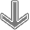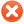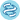Plot optionsQuality control# Use multiple genesUse list of genes Use mean expression of selected genes Use ratio of two genes Filter by median expression
more genes param

# ExampleExample input for multiple genes:
1007_s_at 1
200000_s_at -1
31637_s_at 0.5
40016_g_at -2

Explanation:
first: normal,
second: gene is inverted,
third: gene has weight,
fourth: gene has weight and is inverted

You can also use following format:
1007_s_at, 1
200000_s_at, -1
31637_s_at, 0.5
40016_g_at, -2

 Split patients by --none--lower quartilelower tertilemedianupper tertileupper quartile Auto select best cutoffTrichotomization : --none--T1 vs T3Q1 vs Q4Survival: RFS (n=4934)OS (n=1880)DMFS (n=2767)PPS (n=458)Compute median survival:Follow up threshold: all2 months4 months6 months12 months18 months24 months30 months36 months48 months60 months120 months180 months240 monthsCensore at threshold:## Probe set options

Use
 user selected probe set all probe sets per geneonly JetSet best probe setPlot beeswarm graph of probe distribution:## Restrict analysis to subtypes...

 ER status - IHC: (n=5667) all ER positive (n=3499) ER negative (n=2168) ER status - array: (n=7535)all ER positive (n=5526) ER negative (n=2009) PR status - IHC: (n=3548) all PR positive (n=1559) PR negative (n=1989) HER2 status - array: (n=7535) all HER2 positive (n=1273) HER2 negative (n=6262) Subtype - StGallen: (n=7535)all basal (n=1494) luminal A (n=3511) luminal B (n=2015) HER2+ (n=515)Subtype - PAM50: (n=7535) all each separately basal (n=1671) luminal A (n=2470) luminal B (n=2005) HER2+ (n=1080) normal (n=309) Lymph node status: (n=4994) all Lymph node positive (n=2153) Lymph node negative (n=2829) Grade: (n=4429) all 1 (n=576) 2 (n=1795) 3 (n=2058) TP53 status: (n=660)all mutated (n=272) wild type (n=388) Pietenpol subtype: (n=2041) all basal-like 1 (n=418) basal-like 2 (n=165) immunomodulatory (n=462) mesenchymal (n=382) mesenchymal stem–like (n=201) luminal androgen receptor (n=413)

## Restrict analysis to selected cohorts...

 systemically untreated patients: include (n=1030)exclude (n=3733) patients with following systemic treatment: endocrine therapy:any (n=3851)include (n=1813)exclude (n=2038)tamoxifen only (n=1063) chemotherapy: any (n=4513)include all (n=2342)adjuvant only (n=746)neoadjuvant only (n=1615)exclude all (n=2105) patient cohort similar to SEER prevalencesUse following dataset for the analysis: alleach separatelyE-MTAB-365 (n=537)E-TABM-43 (n=37)GSE11121 (n=200)GSE12093 (n=136)GSE12276 (n=204)GSE1456 (n=159)GSE16391 (n=55)GSE16446 (n=120)GSE16716 (n=47)GSE17705 (n=196)GSE17907 (n=54)GSE18728 (n=61)GSE19615 (n=115)GSE20194 (n=45)GSE20271 (n=96)GSE2034 (n=286)GSE20685 (n=327)GSE20711 (n=90)GSE21653 (n=240)GSE22093 (n=68)GSE25066 (n=507)GSE2603 (n=99)GSE26971 (n=276)GSE29044 (n=79)GSE2990 (n=102)GSE31448 (n=71)GSE31519 (n=67)GSE32646 (n=115)GSE3494 (n=251)GSE36771 (n=107)GSE37946 (n=41)GSE41998 (n=279)GSE42568 (n=121)GSE43358 (n=57)GSE43365 (n=111)GSE45255 (n=139)GSE4611 (n=153)GSE46184 (n=74)GSE48390 (n=81)GSE50948 (n=156)GSE5327 (n=58)GSE58812 (n=107)GSE61304 (n=62)GSE65194 (n=164)GSE6532 (n=82)GSE69031 (n=130)GSE7390 (n=198)GSE76275 (n=265)GSE78958 (n=424)GSE9195 (n=77)

Please note: the generated p value does not include correction for multiple hypothesis testing by default.Please kindly cite following paper to support further development: Győrffy B. Survival analysis across the entire transcriptome identifies biomarkers with the highest prognostic power in breast cancer, Computational and Structural Biotechnology Journal, 2021;19:4101-4109, https://doi.org/10.1016/j.csbj.2021.07.014